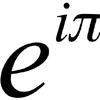#### You may also like### Target Six

Show that x = 1 is a solution of the equation x^(3/2) - 8x^(-3/2) = 7 and find all other solutions.### 8 Methods for Three by One

This problem in geometry has been solved in no less than EIGHT ways by a pair of students. How would you solve it? How many of their solutions can you follow? How are they the same or different? Which do you like best?### An Introduction to Complex Numbers

A short introduction to complex numbers written primarily for students aged 14 to 19.

# Roots and Coefficients

##### Age 16 to 18Challenge Level

If $z_1 z_2 z_3 = 1$ and $z_1 + z_2 + z_3 = \frac{1}{z_1} + \frac{1}{z_2} +\frac{1}{z_3}$ then show that at least one of these numbers must be 1.

Now for the complexity! When are the other numbers real and when are they complex?